Balanced chemical equations What is a balanced chemical equation? A normal chemical equation shows : - the reactants and products, their chemical formulae and their states. A balanced chemical equation, however, shows the proper ratio in which the reactants react and the products form. All chemical reactions obey the "Law of Conservation of Mass" and have the same number of atoms of each element before and after the reaction. A balanced chemical equation, therefore, should have the same number of atoms of each element on each side of the equation. How do you balance a chemical equation? Lets consider our first example. Click on the blue wrting for assistance. Write the chemical equation of the reaction between hydrogen gas and oxygen gas to form liquid water. There are several steps to follow. 1) Write the formulae of the reactants and products separated by an arrow 2) Never change the formulae of reactants and products. 3) Always use coefficients in front of formulae to balance the equation (this step is the most involved) 4) Write the state of each reactant and product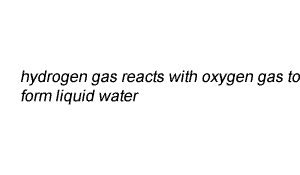Lets see how we derived the coefficients for each reactant and product. When learning to balance camel equations it is best to think in pictures. So on the right the molecules of each reactant and product are shown. It is clear to see that we do not have the same number of atoms of each element on both sides of the equation. So its time to balance the equation. We have two oxygen atoms on the left and one on the right. Clearly this is not right. Step 1 Lets balance for oxygen by adding another water molecule to the right. After step 1, above, we have two oxygen and 4 hydrogen atoms on the right. Step 2 We must now balance for hydrogen atoms by adding another hydrogen molecule on the left. This gives us a balanced chemical equation with equal numbers of each atom on both sides of the equation.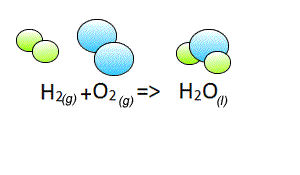Lets consider our second example. Write the chemical equation of the reaction between ethene gas (C2H4) and oxygen gas (O2) to form liquid water(H2O) and carbon dioxide gas (CO2). There are several steps to follow. 1) Write the formulae of the reactants and products separated by an arrow 2) Never change the formulae of reactants and products. 3) Always use coefficients in front of formulae to balance the equation (this step is the most involved) 4) Write the state of each reactant and product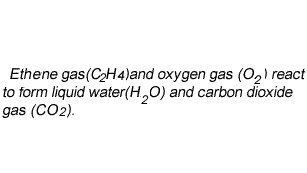Now lets see how we derive the coefficients. To start balancing the equation it is easier to pick an element that appears once on each side of the equation. In this case you can start with either carbon or hydrogen as oxygen appears twice on the products side in both H2O and CO2. Step 1 Let's start with carbon. We have two carbons on the left and one on the right, so lets put two molecules of CO2 on the right. This will balance for carbons. Step 2 Now let's balance for hydrogens. We have four on the left and two on the right, so lets add another H2O molecule to the right side. Now it is time to balance for oxygen. We now have 2 on the left and 6 on the right. Step 3 Balance for oxygens by adding two more molecules of oxygen on the left to make a total of three molecules of oxygen on the left.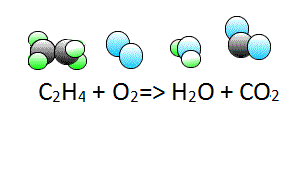Our third example Write the chemical equation of the reaction between liquid ethane (C2H6) and oxygen gas (O2) to form water vapour (H2O) and carbon dioxide gas (CO2). There are several steps to follow. 1) Write the formulae of the reactants and products separated by an arrow 2) Never change the formulae of reactants and products. 3) Always use coefficients in front of formulae to balance the equation (this step is the most involved) 4) Write the state of each reactant and product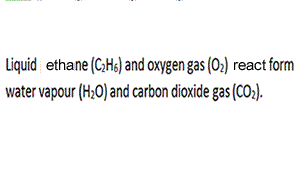Now lets see how we derive the coefficients. To start balancing the equation it is easier to pick an element that appears once on each side of the equation. In this case you can start with either carbon or hydrogen as oxygen appears twice on the products side in both H2O and CO2. Step 1 Let's start with carbon. We have two carbons on the left and one on the right so lets put two molecules of CO2 on the right. This will balance for carbons. Step 2 Now let's balance for hydrogens. We have six hydrogen atoms on the left and two on the right, so lets add another 2 H2O molecules to the right side to make a total of 3 water molecules. Now it is time to balance for oxygen. We now have two on the left and 7 on the right. Step 3 Balance for oxygens by placing three and a half oxygen molecules on the left. Technically this gives us 7 atoms of oxygen on both sides. Step 4 We cannot have half an oxygen molecule so multiply the entire equation by two to remove the fraction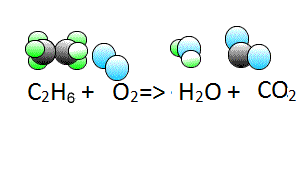Attempt the following. 1) Hydrogen gas(H2) reacts with nitrogen gas(N2) to form ammonia gas (NH3) Solution How did we balance this equation?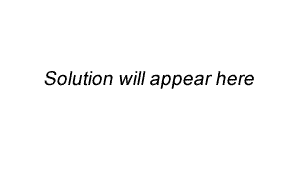2) Propane gas reacts with oxygen to form carbon dioxide gas and water vapour. 3) Hydrochloric acid (HCl) solution and zinc metal (Zn) reacts to form hydrogen gas (H2) and zinc chloride (ZnCl2) solution. Solution 4) Hydrocholoric acid (HCl) solution reacts with solid calcium carbonate (CaCO3) to form calcium chloride (CaCl2)solution, carbon dioxide (CO2) gas and liquid water (H2O). Solution 5) Liquid butane (C4H10) burns in oxygen to form carbon dioxide (CO2) gas and water vapour (H2O). 6) Solid ammonium nitrate (NH4NO3) decomposes according to the unbalanced equation below. NH4NO3(s) => N2O(g) + H2O(g) Solution Click for a link that provides another way to balance chemical equations using fractions and may be of help. Try these exercises. 1) Sr(s) + O2 (g) => SrO(s) 2) HBr (g) + Ca(OH)2 (s) => CaBr2 (s)+ H2O(l) 3) Al(s) + O2(g) => Al2O3(s) 4) C3H6(g) + O2(g) => CO2(g) + H2O(l) 5) Pb(NO3)2(aq) + KI (aq) => PbI2(s) + KNO3(aq) 6) HCl (aq) + Al (s) => AlCl3(aq) + H2(g) 7) H2SO4(aq) + CaCO3(s) => CO2(g) + H2O(l) + CaSO4(aq) Solutions Continue with balancing equations involving ionic compounds